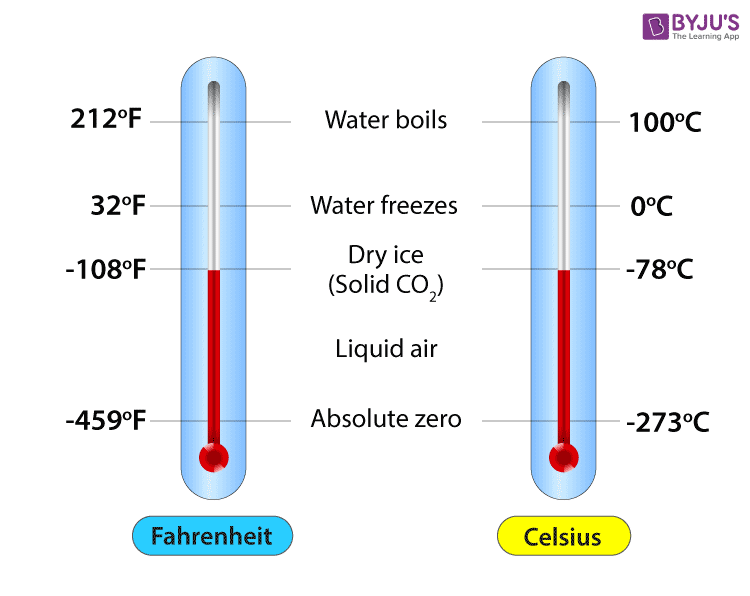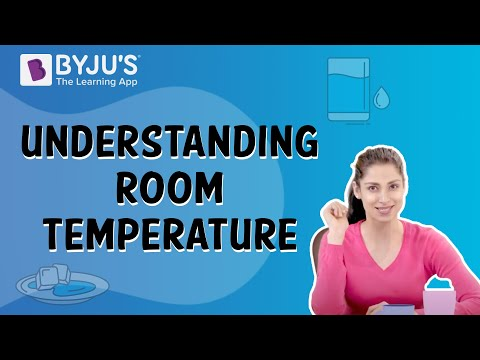Checkout JEE MAINS 2022 Question Paper Analysis : Checkout JEE MAINS 2022 Question Paper Analysis :

# Relation Between Celsius And Fahrenheit

Celsius and Fahrenheit are two scales used to measure temperature. The temperature in the centigrade scale will be expressed in degrees Celsius. The temperature in the Fahrenheit scale will be expressed in degrees Fahrenheit. The relation between Celsius and Fahrenheit is proportional. Both have a different freezing point of water and both follow the varied unit difference between each scale.

## Celsius to Fahrenheit

The conversion from Celsius to Fahrenheit can be mathematically expressed as-

 $$\begin{array}{l}F=(\frac{9}{5}\times C)+32\end{array}$$

Where,

• F is the temperature in Fahrenheit.
• C is the temperature on the Centigrade scale.

## Fahrenheit to Celsius

The conversion from Fahrenheit to Celsius can be mathematically expressed as-

 $$\begin{array}{l}C=\frac{5}{9}(F-32)\end{array}$$

Where,

• F is the temperature in Fahrenheit.
• C is the temperature on the Centigrade scale.

Examples:

### 1 degrees Celsius to Fahrenheit

$$\begin{array}{l}F=(\frac{9}{5}\times 1)+32\end{array}$$
33.8 °F

### 1 Fahrenheit to Celsius

$$\begin{array}{l}C=\frac{5}{9}(1-32)\end{array}$$
-17.2222 °C

### Units of Celsius and Fahrenheit

The units of temperature scale are useful in differentiating temperatures from one scale to another.

• The temperature on the Celsius scale is denoted by °C
• The temperature on the Fahrenheit scale is denoted by °F

## Relation between °C and °FThe relation between Celsius and Fahrenheit is direct. Celsius is directly proportional to Fahrenheit, which implies that –

• When the temperature in Celsius scale increases, its equivalent Fahrenheit temperature will also be high.
• When the temperature in Celsius scale decreases, its equivalent Fahrenheit temperature will also be low.

Both scales have their own thermometer for measurements. Fahrenheit scale is proposed by taking 32 °F as a freezing point of water and 212 °F as a boiling point of water. Whereas in the Celsius scale 0 °C is the freezing point of water and 100 °C is the boiling point of water.

Hope you understood the relation and conversion between the Celsius and Fahrenheit scale of temperature.

Physics Related Topics:

Stay tuned to BYJU’S for more such interesting articles. Also, register to “BYJU’S-The Learning App” for loads of interactive, engaging physics-related videos and an unlimited academic assist.

## Understanding Room Temperature## Frequently Asked Questions – FAQs

### What is the formula to convert from Celsius to Fahrenheit?

The formula to convert from Celsius to Fahrenheit is F= (9/5 x C) + 32.

### What is the formula to convert from Fahrenheit to Celsius?

The formula to convert from Fahrenheit to Celsius is C = 5/9(F – 32).

### What is the SI unit of temperature?

The SI unit of temperature is Kelvin.

### What are the other units of temperature?

Other units of temperature are Rømer, Rankine, Réaumur, Delisle, and Newton.

### What are the three common temperature scales?

The three common temperature scales are
• Celsius
• Fahrenheit
• Kelvin
• Test Your Knowledge On Relation Between Celsius And Fahrenheit!